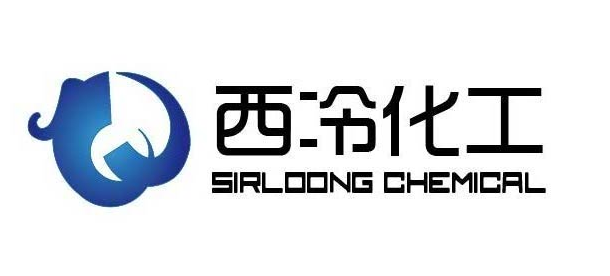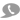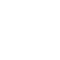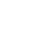15

18653007758m=碳原子数

n=氢原子数

p=氯原子数

1. 第一个数字是碳原子减一。

2. 左起第二位氢原子数加一。

3. 制冷剂命名不考虑氯。

4. 左数第三位的氟原子。

F

|

Cl-C-Cl

|

F

n=0,

p=2,

q=2,

=R (m-1)(n+1)q=R (1-1)(0+1)2=R12

F F

| |

| |

F F

n=0,

p=2,

q=4,

=R (m-1)(n+1)q=R (2-1)(0+1)4=R114

新闻分类